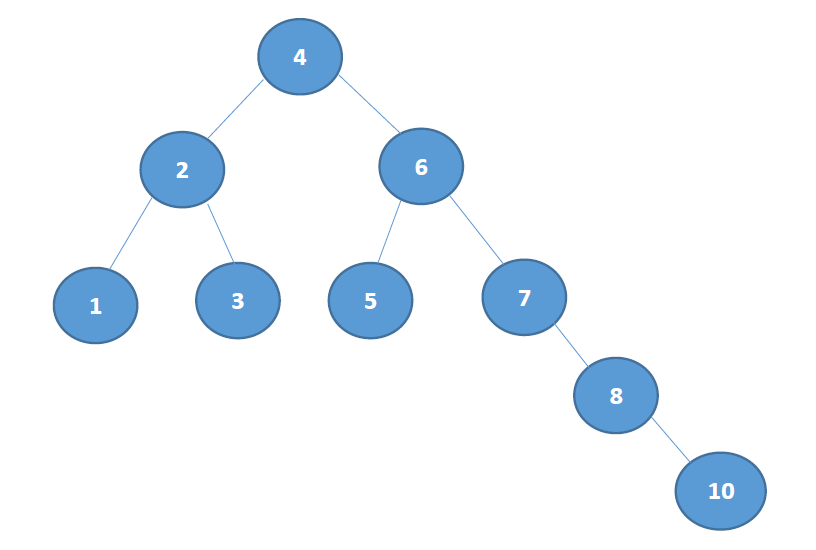# ********2019-2020-1 《数据结构与面向对象程序设计》第九周学习总结

## 教材学习内容总结

• 树的特点：

• 每个结点有零个或多个子结点；没有父结点的结点称为根结点；每一个非根结点有且只有一个父结点；除了根结点外，每个子结点可以分为多个不相交的子树
• 二叉树的前序、中序、后序、层序遍历（非递归方法）：• 前序遍历：4 2 1 3 6 5 7 8 10

• 中序遍历：1 2 3 4 5 6 7 8 10

• 后序遍历：1 3 2 5 10 8 7 6 4

• 层序遍历：4 2 6 1 3 5 7 8 10

public void preOrder(BinaryNode<AnyType> Node)
{
if (Node != null)
{
System.out.print(Node.element + " ");
preOrder(Node.left);
preOrder(Node.right);
}
}


public void midOrder(BinaryNode<AnyType> Node)
{
if (Node != null)
{
midOrder(Node.left);
System.out.print(Node.element + " ");
midOrder(Node.right);
}
}



public void posOrder(BinaryNode<AnyType> Node)
{
if (Node != null)
{
posOrder(Node.left);
posOrder(Node.right);
System.out.print(Node.element + " ");
}
}


public void levelOrder(BinaryNode<AnyType> Node) {
if (Node == null) {
return;
}

int depth = depth(Node);

for (int i = 1; i <= depth; i++) {
levelOrder(Node, i);
}
}

private void levelOrder(BinaryNode<AnyType> Node, int level) {
if (Node == null || level < 1) {
return;
}

if (level == 1) {
System.out.print(Node.element + "  ");
return;
}

// 左子树
levelOrder(Node.left, level - 1);

// 右子树
levelOrder(Node.right, level - 1);
}

public int depth(BinaryNode<AnyType> Node) {
if (Node == null) {
return 0;
}

int l = depth(Node.left);
int r = depth(Node.right);
if (l > r) {
return l + 1;
} else {
return r + 1;
}
}



public void preOrder1(BinaryNode<AnyType> Node)
{
Stack<BinaryNode> stack = new Stack<>();
while(Node != null || !stack.empty())
{
while(Node != null)
{
System.out.print(Node.element + "   ");
stack.push(Node);
Node = Node.left;
}
if(!stack.empty())
{
Node = stack.pop();
Node = Node.right;
}
}
}



 public void levelOrder1(BinaryNode<AnyType> Node) {
if (Node == null) {
return;
}

BinaryNode<AnyType> binaryNode;

while (queue.size() != 0) {
binaryNode = queue.poll();

System.out.print(binaryNode.element + "  ");

if (binaryNode.left != null) {
queue.offer(binaryNode.left);
}
if (binaryNode.right != null) {
queue.offer(binaryNode.right);
}
}
}

• 二叉树的重要性质：
• 在二叉树的第i层上最多有2 i-1 个节点
• 二叉树中如果深度为k,那么最多有2k-1个节点。(k>=1）
• n0=n2+1 n0表示度数为0的节点 n2表示度数为2的节点
• 在完全二叉树中，具有n个节点的完全二叉树的深度为[log2n]+1，其中[log2n]+1是向下取整。
• 若对含 n 个结点的完全二叉树从上到下且从左至右进行 1 至 n 的编号，则对完全二叉树中任意一个编号为 i 的结点：
• (1) 若 i=1，则该结点是二叉树的根，无双亲, 否则，编号为 [i/2] 的结点为其双亲结点;
• (2) 若 2i>n，则该结点无左孩子， 否则，编号为 2i 的结点为其左孩子结点；
• (3) 若 2i+1>n，则该结点无右孩子结点， 否则，编号为2i+1 的结点为其右孩子结点。

## 代码调试中的问题和解决过程

ForEach、迭代器、for循环性能比较

## 上周考试错题总结

1.In an ideal implementations of a stack and a queue, all operations are ______________________ .

A . O(1)

B . O(n)

C . O(n log n)

D . O(n2)

E . it depends on the operation

2.If a binary search tree is not __________, it may be less efficient than a linear structure.

A . complete

B . empty

C . balanced

D . None of the above

3.It is possible to implement a stack and a queue in such a way that all operations take a constant amount of time.

A .true

B .false

4.In a circular array-based implementation of a queue, the elements must all be shifted when the dequeue operation is called.

A .true

B .false

## 结对及互评

### 点评

• 博客中值得学习的：
• 教材学习内容概括行强，简介明了。
• 有自己动手打新代码，加入了很多个人的理解。
• 内容很充实，很用心，比上次进步了很多。
• 博客格式正确，运用了很多不同的方法，排版精美。
• 希望能在课本内容总结以及问题&解决过程中加入自己的思考，使博客内容更加充实。
基于评分标准，我给本博客打分12分：
得分情况如下：
正确使用Markdown语法（加1分）
模板中的要素齐全（加1分）
教材学习中的问题和解决过程（加2分）
代码调试中的问题和解决过程（加2分）
其他加分（加6分）
进度条中记录学习时间与改进情况（1）
感想，体会不假大空（1）
有动手写新代码（1）
错题学习深入（1）
点评认真，能指出博客和代码中的问题（1）
结对学习情况真实可信（1）

• 本周结对学习情况：

## 参考资料

| 代码行数（新增/累积） | 博客量（新增/累积）|学习时间（新增/累积）|重要成长
---|---|---|---|---

posted @ 2019-11-19 20:57  Mr.Stark丿  阅读(226)  评论(0编辑  收藏  举报